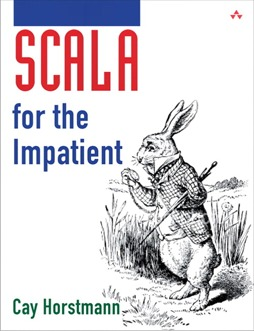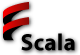# Scala for the Impatient

## Scala Basics# Why Scala?• Object-oriented and functional.
• Statically typed.
• Scalable from simple scripts to sophisticated (but still easy-to-use) libraries.
• Invented by Martin Odersky at EPFL.
• Works with Java VM tools/libraries/infrastructure.
• Used for big-iron projects in industry.
• Pragmatic choice as successor to Java (whose evolution has slowed to a crawl).
• Expands your mind and makes you think differently about programming in any language.

# Scala Levels of Expertise

These lessons are A1 unless noted otherwise.

Application Programmer Library Designer Overall Scala Level
Beginning A1 Beginning
Intermediate A2 Junior L1 Intermediate
Expert L3 Expert

# Use Scala worksheets

Live demo of worksheets

# Declaring Variables

• Types `Int`, `Double`, `Boolean`, `String`.
• Arithmetic operators `+ - * / %` like in Java.
• Variable type is inferred:
`val answer = 8 * 5 + 2 // answer is an Int`
• Can specify type after the name:
`var greeting: String = null`
• `val`: immutable, `var`: mutable.
• Use `val` whenever you can.
• Semicolons at the end of a statement are optional.

# Commonly Used Types

• `Int`, `Double`, `Byte`, `Char`, `Short`, `Long`, `Float`, `Boolean`.
• Everything is an object:
`1.to(10) // Apply to method to 1, returns Range(1, 2, 3, 4, 5, 6, 7, 8, 9, 10)`
• The `to` method is actually defined in `RichInt`.
• `java.lang.String` is available in Scala.
• Augmented by > 100 methods in `StringOps`:
```"Hello".intersect("World") // Yields "lo"
```
• Big numbers are actually usable:
```val x: BigInt = 1234567890
x * x * x // Yields 1881676371789154860897069000```
• Infix notation:
```1 to 10 // Same as 1.to(10)
1 + 10 // Same as 1.+(10)```
• The `++`, `--` operators do not exist in Scala.
• Use `+=` instead: `counter += 1`.

# Calling Functions and Methods

• Scala has both functions and methods:
```import scala.math._
sqrt(2) // A function
BigInt.probablePrime(100, scala.util.Random) // A method
```
• Methods without parameters usually don't use `()`:
```"Hello".distinct
```
Rule of thumb: `()` only required for mutators
• Common to use `(arg)` for methods that are similar to function calls:
`"Hello"(4) // Yields 'o'`
• Provided by `apply` method:
`"Hello".apply(4) // same as "Hello"(4)`

• Use the filter.
• Look inside companion objects (O = Object, C = Class).
• Look at packages (`scala.math`), `RichXxx`, `StringOps`.
• Methods can have function parameters:
`def count(p: (Char) => Boolean): Int`
• Lots of classes. Use your intuition. `Range`, `Seq[Char]` mean what you think they do.
• Lots of methods.
• Don't freak out when you see the occasional indecipherable incantation:
```def scan[B >: Char, That](z: B)(op: (B, B) ⇒ B)(implicit cbf:
CanBuildFrom[String, B, That]): That ```

# Lab# Part 1: The Scala Worksheet

1. Start the Scala IDE and make a new Scala project: File → New → Scala Project.
Give a name `Lesson1`.
Right-click on the project in the Package Explorer, then select New → Scala Worksheet. Call it `Sheet1`.
Select Project from the menu and uncheck the Build Automatically checkbox.
Make a new line before the `}` . Type `6 * 7` and then save (Ctrl+S/⌘+S). What do you get?
2. Edit the line to read `val a = 6 * 7` and save. What do you get?
3. Add `a`. What do you get?
4. Add `a = 43`. What do you get? Why?
5. Remove that line and add `val b;` (This time with a semicolon.) What do you get? Why?
6. Change the line to `val b: BigInt = 6 * 7`. What do you get?
7. Add `b.pow(1000)`. What do you get?

# Part 2: Functions

1. Type `import scala.math._` just above the `}`.
2. Below the import statement, type:
```sqrt(10)
```
What do you get?
3. Type `1.to(10)`. What do you get?
4. Type `1.to(10).map(sqrt(_))`. What do you get?
5. Type `6.*(7)`. What do you get? Why?

Click on `Int`, then click on the large C icon. Watch it turn into an O.
What is the Scala analog of `java.lang.Integer.MAX_VALUE`?
Click on `StringOps`.
Read the description of `distinct`.
What do you expect the result of `"Mississippi".distinct` to be?
4. Read the description of `permutations`.
What do you expect the result of `"Rhine".permutations` to be?
5. That's not so good. Add `.toArray`. Now what do you get?
6. What do you get for `"ABC".sum`? Why? (Hint: Try `'A'.toInt` and `"ABC".sum.toInt`)